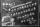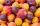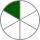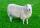# Calculator fractional exponents

The calculator performs basic and advanced operations with fractions, expressions with fractions combined with integers, decimals, and mixed numbers. Also shows detailed step-by-step information about fraction calculation procedure. Solve problems with two, three or more fractions and numbers in one expression.

### 16 ^ 1/2 = 4

Spelled result in words is four.

### How do you solve fractions step by step?

1. Exponentiation: 16 ^ 12 = 4

In words - sixteen one half = four.

#### Rules for expressions with fractions:

Fractions - use the slash “/” between the numerator and denominator, i.e. for five-hundredths enter 5/100. If you are using mixed numbers be sure to leave a single space between the whole number and fraction part.
The slash separates the numerator (number above a fraction line) and denominator (number below).

Mixed numerals (mixed fractions or mixed numbers) write as non-zero integer separated by one space and fraction i.e., 1 2/3 (having the same sign). An example of a negative mixed fraction: -5 1/2.
Because slash is both signs for fraction line and division, we recommended use colon (:) as operator of division fractions i.e., 1/2 : 3.

Decimals (decimal numbers) enter with a decimal point . and they are automatically converted to fractions - i.e. 1.45.

Colon : and slash / is the symbol of division. Can be used to divide mixed numbers 1 2/3 : 4 3/8 or can be used for write complex fractions i.e. 1/2 : 1/3.
An asterisk * or × is the symbol for multiplication.
Plus + is addition, minus sign - is subtraction and ()[] is mathematical parentheses.
The exponentiation/power symbol is ^ - for example: (7/8-4/5)^2 = (7/8-4/5)2

Calculator follows well-known rules for order of operations. Most common mnemonics for remembering this order of operations are:
PEMDAS - Parentheses, Exponents, Multiplication, Division, Addition, Subtraction.
BEDMAS - Brackets, Exponents, Division, Multiplication, Addition, Subtraction
BODMAS - Brackets, Of or Order, Division, Multiplication, Addition, Subtraction.
GEMDAS - Grouping Symbols - brackets (){}, Exponents, Multiplication, Division, Addition, Subtraction.
Be careful, always do multiplication and division before addition and subtraction. Some operators (+ and -) and (* and /) has the same priority and then must evaluate from left to right.

## Fractions in word problems:

• In fractionsAn ant climbs 2/5 of the pole on the first hour and climbs 1/4 of the pole on the next hour. What part of the pole does the ant climb in two hours?
• Scouts 44/7 of the students in a school are boys. If 3/8 of the boys are scouts, how many scouts are there in a school of 1878 students?
• Unknown numberI think the number - its sixth is 3 smaller than its third.
• Unknown number 6Determine x if 1/6 of x is equal to 2/5 of the number 24.
• InqualitySolve inequality: 3x + 6 > 14
• HomeworkIn the crate are 18 plums, 27 apricot and 36 nuts. How many pieces of fruit left in the crate when Peter took 8 ninth: 1. nuts 2. apricots 3. fruit 4. drupe
• Tallest and shortestJenn is the tallest on the team. He is 1 1/2 times as tall as the shortest girl whom is 4 1/4 feet tall. How tall is Jebb?
• Unit rateFind unit rate: 6,840 customers in 45 days
• DividendsThe three friends divided the win by the invested money. Karlos got three eighths John 320 permille and the rest got Martin. Who got the most and which the least?
• Reciprocal equation 3Solve reciprocal equation: 1/2 + 2/3=1/x
• Equivalent fractionsAre these two fractions equivalent -4/9 and 11/15?
• One sixthHow many sixths are two thirds?
• The farmerThe farmer had 140 sheep. For the next year, she decided to change the number of sheep in ratio 10: 7. How many sheep will he have then?